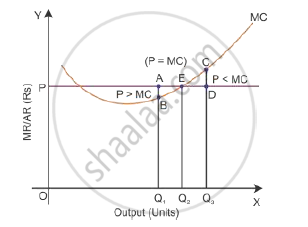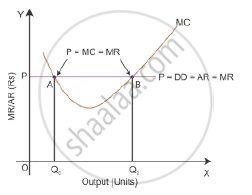# Explain the Conditions of a Producer'S Equilibrium in Terms of Marginal Cost and Marginal Revenue. Use Diagram. - Economics

Explain the conditions of a producer's equilibrium in terms of marginal cost and marginal revenue. Use diagram.

Explain the conditions of producer’s equilibrium in terms of marginal revenue and marginal cost.

#### Solution

The producer’s equilibrium refers to the situation in which he maximises his profits. A producer strikes an equilibrium when two conditions are satisfied.

i. MR = MC
ii. MC is rising or the MC curve cuts the MR curve from below.

MR, MC Schedule and Producer’s Equilibrium:

 Output MR MC 1 10 8 2 10 7 3 10 6 4 10 8 5 10 10 6 10 13

Here, it is assumed that price (AR) is constant, so that MR is constant, i.e. = Rs 10 under perfect competition. This table indicates that the two conditions of equilibrium are satisfied only when 5 units of output are produced. It is here that (i) MR = MC = Rs 10 and (ii) MC is rising.
Equilibrium is not struck when MR > MC. In such a situation, producing an additional unit would add more to TR than to TC. This implies that the gap between TR and TC tends to widen or that profits are still to be maximised.

Condition 1:
i. If MR > MC:
Suppose OQ1 is the output level at the price AQ1 and the marginal cost is BQ1, then it would be AQ1 > BQ1. Here, OQ1 is not the level of output at which the profit is maximised. So, the firm can increase its profit by increasing the production to the OQ2 level of output.ii. If Price (MR) < MC
Suppose OQ3 is the output level at the price DQ3 and the marginal cost is CQ3, then it would be DQ3 < CQ3. Here, OQ3 is not the level of output at which the profit is maximised. So, the
firm can increase its profit by decreasing its output level to OQ2. Thus, the firm’s equilibrium level of output to maximise output is that MR = MC and MC should be rising at the point of intersection with MR.

Condition 2:
In the given diagram, the MC curve intersects the price line (or MR) at two points—A and B. Here Condition 1 of profit maximisation MR = MC is satisfied at these two points. Next, let us consider Condition 2.i. Intersection point at A
At intersection point A, price is equal to MC but MC is falling, and it is a downward-sloping curve. If the output is increased more than the OQ0 level, then the price is more than MC. This means that the firm can increase the production more than the OQ0 level of output to maximise profit.

ii. Intersection point at B
At intersection point B, if the output is increased more than output OQ2, the price is more than MC. This states that the firm can increase the production more than the OQ2 level of output to maximise profit. While if the firm produces higher level of output than OQ2, then the price is less than MC. This clearly states that high profit is possible by decreasing the output level to OQ2. Thus, Point B is the producer’s equilibrium and OQ2 is the output level to maximise profit by satisfying the two necessary conditions (i) Price = MC and (ii) the MC curve is rising

Concept: Concept of Producer's Equilibrium
Is there an error in this question or solution?
2011-2012 (March) Delhi Set 1

Share